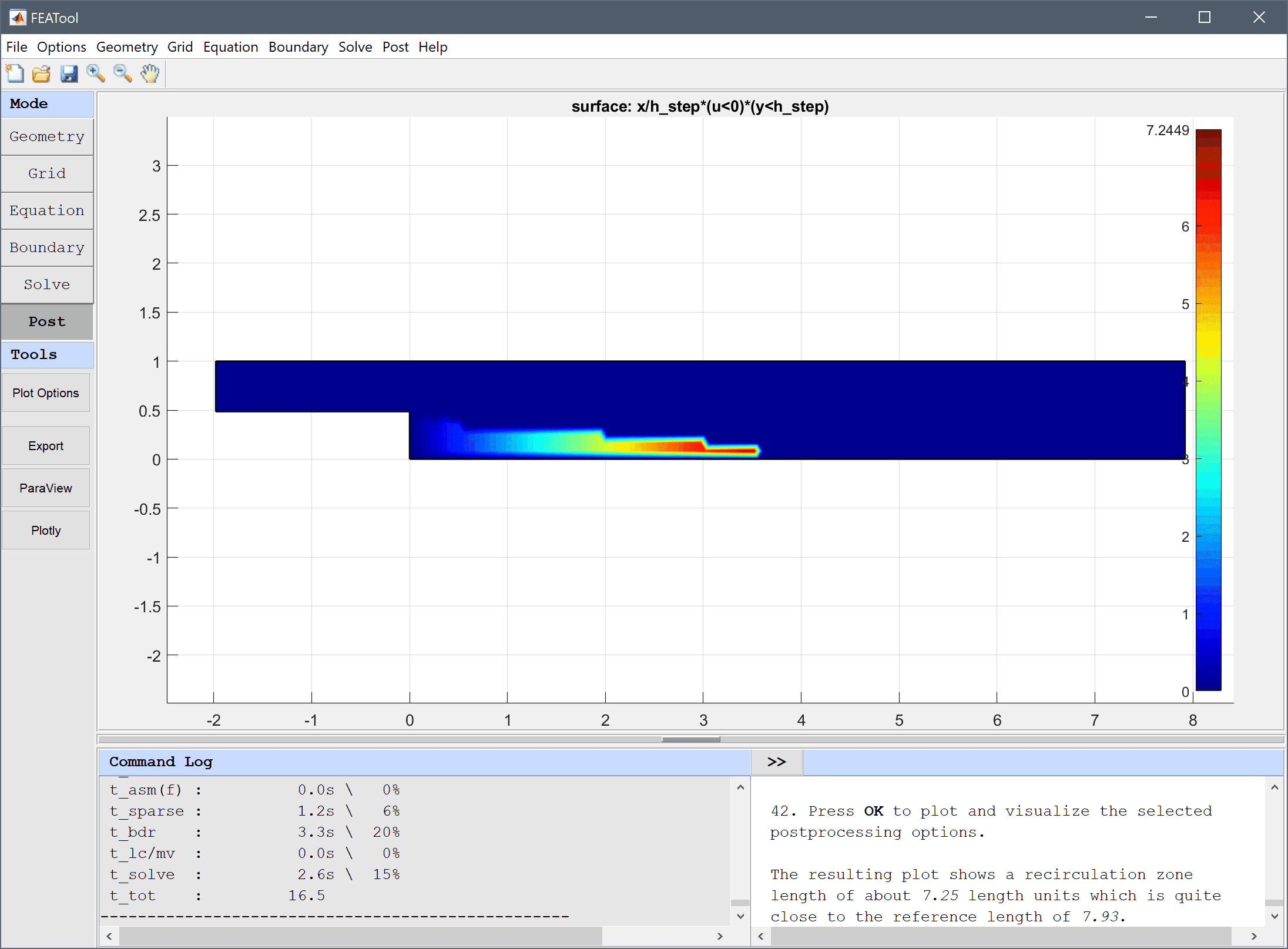# Flow Over a Backwards Facing Step## Model Data

Type: fluid dynamics

Physics Modes: navier stokes equations

Keywords: validation

Flow over a backwards facing step is a classic computational fluid dynamics test problem which is used extensively for validation of simulation codes. The test problem essentially consists of studying how a fully developed flow profile reacts to a sudden expansion in a channel. The expansion will cause a break in the flow and a recirculation or separation zone will form. To measure and compare results the resulting length of the recirculation or separation zone is used.

The stationary incompressible Navier-Stokes equations are applied with simulation parameters corresponding to a Reynolds number, Re = 389. The inlet velocity is given as uinlet = 4umax (y-hstep)(1-y)/hinlet2 where hinlet is the channel height, hstep the expansion step height, and umax = 1 the maximum velocity. No-slip zero velocity conditions are applied to all solid walls, and a suitable outflow condition must also be applied. The reference recirculation zone length found in the references ,  is estimated to be 7.93 length units (fraction of the step height).

This model is available as an automated tutorial by selecting Model Examples and Tutorials… > Fluid Dynamics > Flow Over a Backwards Facing Step from the File menu, and also as the MATLAB simulation m-script example ex_navierstokes4. Step-by-step tutorial instructions to set up and run this model are linked below.P.M. Gresho and R.L. Sani, Incompressible Flow and the Finite Element Method, Volume 1 & 2, John Wiley & Sons, New York, 2000.

 A. Rose and B. Simpson: Laminar, Constant-Temperature Flow Over a Backward Facing Step, 1st NAFEMS Workbook of CFD Examples, Glasgow, UK, 2000.